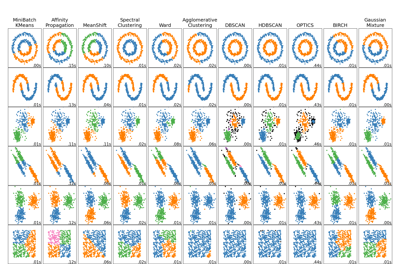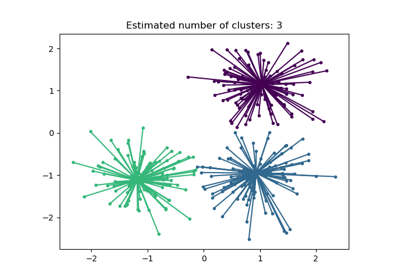# sklearn.cluster.AffinityPropagation¶

class sklearn.cluster.AffinityPropagation(*, damping=0.5, max_iter=200, convergence_iter=15, copy=True, preference=None, affinity='euclidean', verbose=False, random_state=None)[source]

Perform Affinity Propagation Clustering of data.

Read more in the User Guide.

Parameters:
dampingfloat, default=0.5

Damping factor in the range [0.5, 1.0) is the extent to which the current value is maintained relative to incoming values (weighted 1 - damping). This in order to avoid numerical oscillations when updating these values (messages).

max_iterint, default=200

Maximum number of iterations.

convergence_iterint, default=15

Number of iterations with no change in the number of estimated clusters that stops the convergence.

copybool, default=True

Make a copy of input data.

preferencearray-like of shape (n_samples,) or float, default=None

Preferences for each point - points with larger values of preferences are more likely to be chosen as exemplars. The number of exemplars, ie of clusters, is influenced by the input preferences value. If the preferences are not passed as arguments, they will be set to the median of the input similarities.

affinity{‘euclidean’, ‘precomputed’}, default=’euclidean’

Which affinity to use. At the moment ‘precomputed’ and euclidean are supported. ‘euclidean’ uses the negative squared euclidean distance between points.

verbosebool, default=False

Whether to be verbose.

random_stateint, RandomState instance or None, default=None

Pseudo-random number generator to control the starting state. Use an int for reproducible results across function calls. See the Glossary.

New in version 0.23: this parameter was previously hardcoded as 0.

Attributes:
cluster_centers_indices_ndarray of shape (n_clusters,)

Indices of cluster centers.

cluster_centers_ndarray of shape (n_clusters, n_features)

Cluster centers (if affinity != precomputed).

labels_ndarray of shape (n_samples,)

Labels of each point.

affinity_matrix_ndarray of shape (n_samples, n_samples)

Stores the affinity matrix used in fit.

n_iter_int

Number of iterations taken to converge.

n_features_in_int

Number of features seen during fit.

New in version 0.24.

feature_names_in_ndarray of shape (n_features_in_,)

Names of features seen during fit. Defined only when X has feature names that are all strings.

New in version 1.0.

AgglomerativeClustering

Recursively merges the pair of clusters that minimally increases a given linkage distance.

FeatureAgglomeration

Similar to AgglomerativeClustering, but recursively merges features instead of samples.

KMeans

K-Means clustering.

MiniBatchKMeans

Mini-Batch K-Means clustering.

MeanShift

Mean shift clustering using a flat kernel.

SpectralClustering

Apply clustering to a projection of the normalized Laplacian.

Notes

For an example, see examples/cluster/plot_affinity_propagation.py.

The algorithmic complexity of affinity propagation is quadratic in the number of points.

When the algorithm does not converge, it will still return a arrays of cluster_center_indices and labels if there are any exemplars/clusters, however they may be degenerate and should be used with caution.

When fit does not converge, cluster_centers_ is still populated however it may be degenerate. In such a case, proceed with caution. If fit does not converge and fails to produce any cluster_centers_ then predict will label every sample as -1.

When all training samples have equal similarities and equal preferences, the assignment of cluster centers and labels depends on the preference. If the preference is smaller than the similarities, fit will result in a single cluster center and label 0 for every sample. Otherwise, every training sample becomes its own cluster center and is assigned a unique label.

References

Brendan J. Frey and Delbert Dueck, “Clustering by Passing Messages Between Data Points”, Science Feb. 2007

Examples

>>> from sklearn.cluster import AffinityPropagation
>>> import numpy as np
>>> X = np.array([[1, 2], [1, 4], [1, 0],
...               [4, 2], [4, 4], [4, 0]])
>>> clustering = AffinityPropagation(random_state=5).fit(X)
>>> clustering
AffinityPropagation(random_state=5)
>>> clustering.labels_
array([0, 0, 0, 1, 1, 1])
>>> clustering.predict([[0, 0], [4, 4]])
array([0, 1])
>>> clustering.cluster_centers_
array([[1, 2],
[4, 2]])


Methods

 fit(X[, y]) Fit the clustering from features, or affinity matrix. fit_predict(X[, y]) Fit clustering from features/affinity matrix; return cluster labels. get_params([deep]) Get parameters for this estimator. Predict the closest cluster each sample in X belongs to. set_params(**params) Set the parameters of this estimator.
fit(X, y=None)[source]

Fit the clustering from features, or affinity matrix.

Parameters:
X{array-like, sparse matrix} of shape (n_samples, n_features), or array-like of shape (n_samples, n_samples)

Training instances to cluster, or similarities / affinities between instances if affinity='precomputed'. If a sparse feature matrix is provided, it will be converted into a sparse csr_matrix.

yIgnored

Not used, present here for API consistency by convention.

Returns:
self

Returns the instance itself.

fit_predict(X, y=None)[source]

Fit clustering from features/affinity matrix; return cluster labels.

Parameters:
X{array-like, sparse matrix} of shape (n_samples, n_features), or array-like of shape (n_samples, n_samples)

Training instances to cluster, or similarities / affinities between instances if affinity='precomputed'. If a sparse feature matrix is provided, it will be converted into a sparse csr_matrix.

yIgnored

Not used, present here for API consistency by convention.

Returns:
labelsndarray of shape (n_samples,)

Cluster labels.

get_params(deep=True)[source]

Get parameters for this estimator.

Parameters:
deepbool, default=True

If True, will return the parameters for this estimator and contained subobjects that are estimators.

Returns:
paramsdict

Parameter names mapped to their values.

predict(X)[source]

Predict the closest cluster each sample in X belongs to.

Parameters:
X{array-like, sparse matrix} of shape (n_samples, n_features)

New data to predict. If a sparse matrix is provided, it will be converted into a sparse csr_matrix.

Returns:
labelsndarray of shape (n_samples,)

Cluster labels.

set_params(**params)[source]

Set the parameters of this estimator.

The method works on simple estimators as well as on nested objects (such as Pipeline). The latter have parameters of the form <component>__<parameter> so that it’s possible to update each component of a nested object.

Parameters:
**paramsdict

Estimator parameters.

Returns:
selfestimator instance

Estimator instance.

## Examples using sklearn.cluster.AffinityPropagation¶Comparing different clustering algorithms on toy datasets

Comparing different clustering algorithms on toy datasetsDemo of affinity propagation clustering algorithm

Demo of affinity propagation clustering algorithm